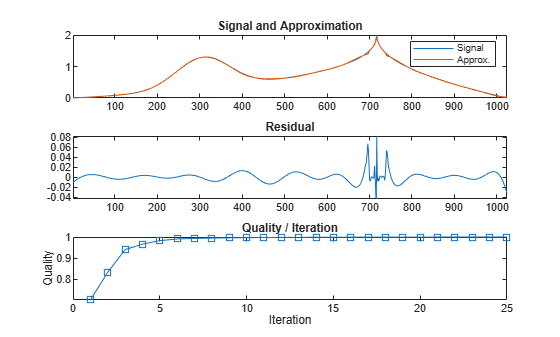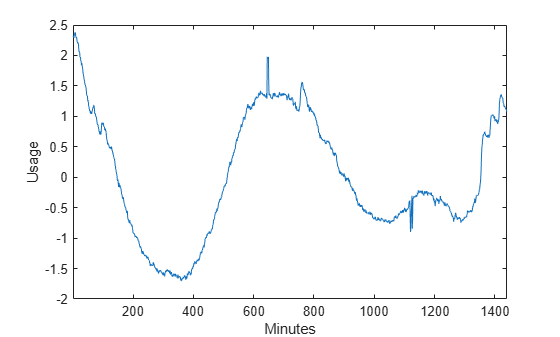# wmpalg

(Not recommended) Matching pursuit

The `wmpalg` function no longer supports plotting and is no longer recommended. See Compatibility Considerations.

## Syntax

``YFIT = wmpalg(MPALG,Y,MPDICT)``
``````[YFIT,R] = wmpalg(___)``````
``````[YFIT,R,COEFF] = wmpalg(___)``````
``````[YFIT,R,COEFF,IOPT] = wmpalg(___)``````
``````[YFIT,R,COEFF,IOPT,QUAL] = wmpalg(___)``````
``````[YFIT,R,COEFF,IOPT,QUAL,X] = wmpalg(___)``````
``````[YFIT,R,COEFF,IOPT,QUAL,X] = wmpalg(___,Name=Value)``````

## Description

````YFIT = wmpalg(MPALG,Y,MPDICT)` returns an adaptive greedy approximation, `YFIT`, of the input signal, `Y`, in the dictionary, `MPDICT`. The adaptive greedy approximation uses the matching pursuit algorithm, `MPALG`. The dictionary, `MPDICT`, is typically an overcomplete set of vectors.```
``````[YFIT,R] = wmpalg(___)``` returns the residual, `R`, which is the difference vector between `Y` and `YFIT` at the termination of the matching pursuit.```
``````[YFIT,R,COEFF] = wmpalg(___)``` returns the expansion coefficients, `COEFF`. The number of expansion coefficients depends on the number of iterations in the matching pursuit.```
``````[YFIT,R,COEFF,IOPT] = wmpalg(___)``` returns the column indices of the retained atoms, `IOPT`. The length of `IOPT` equals the length of `COEFF` and is determined by the number of iterations in the matching pursuit.```
``````[YFIT,R,COEFF,IOPT,QUAL] = wmpalg(___)``` returns the proportion of retained signal energy, `QUAL`, for each iteration of the matching pursuit. `QUAL` is the ratio of the ℓ2 squared norm of the expansion coefficient vector, `COEFF`, to the ℓ2 squared norm of the input signal, `Y`. ```
``````[YFIT,R,COEFF,IOPT,QUAL,X] = wmpalg(___)``` returns the normalized dictionary, `X`. `X` contains the unit vectors in the ℓ2 norm corresponding to the columns of `MPDICT`.```

example

``````[YFIT,R,COEFF,IOPT,QUAL,X] = wmpalg(___,Name=Value)``` returns an adaptive greedy approximation with additional options specified by one or more `Name=Value` arguments.```

## Examples

collapse all

Approximate the `cuspamax` signal with the dictionary using orthogonal matching pursuit.

Use a dictionary consisting of `sym4` wavelet packets and the DCT-II basis.

```load cuspamax yfit = wmpalg('OMP',cuspamax,'lstcpt',{{'wpsym4',2},'dct'}); plot(cuspamax,'k') hold on plot(yfit,'linewidth',2) hold off legend('Original Signal','Matching Pursuit')```Obtain the expansion coefficients in the dictionary, the column indices of the selected dictionary atoms, and the proportion of retained signal energy.

Specify a dictionary consisting of `sym4` wavelet packets and the DCT-II basis. Approximate the `cuspamax` signal with the dictionary using orthogonal matching pursuit.

```load cuspamax; [yfit,r,coeff,iopt,qual] = wmpalg('OMP',cuspamax,... 'lstcpt',{{'wpsym4',2},'dct'});```

This example shows how to set the maximum number of iterations of the orthogonal matching pursuit to 50.

```load cuspamax [yfit,r,coeff,iopt,qual] = wmpalg('OMP',cuspamax,... 'lstcpt',{{'wpsym4',1},{'wpsym4',2},'dct'},... 'itermax',50);```

This example shows how to allow for a suboptimal choice in the update of the orthogonal matching pursuit.

`load cuspamax`

Approximate the signal using weak orthogonal matching pursuit. Relax the requirement to be 0.8 times the optimal assignment.

```[yfit,r,coeff,iopt,qual] = wmpalg('WMP',cuspamax,... 'lstcpt',{{'wpsym4',1},{'wpsym4',2},'dct'},... 'wmpcfs',0.8);```

Plot the signal, approximation, residual, and the proportion of retained signal energy for each iteration in the matching pursuit result.

```subplot(3,1,1) plot(cuspamax) hold on plot(yfit) hold off legend('Signal','Approx.') title('Signal and Approximation') axis tight subplot(3,1,2) plot(r) title('Residual') axis tight subplot(3,1,3) plot(qual,'s-') title('Quality / Iteration') ylabel('Quality') xlabel('Iteration')```Obtain a matching pursuit of electricity consumption measured every minute over a 24-hour period.

Load and plot data. The data shows electricity consumption sampled every minute over a 24-hour period. Because the data is centered, the actual usage values are not interpretable.

```load elec35_nor; y = signals(32,:); plot(y) xlabel('Minutes') ylabel('Usage') set(gca,'xlim',[1 1440])```Specify a dictionary for matching pursuit consisting of the Daubechies' extremal-phase wavelet with 2 vanishing moments at level 2, the Daubechies' least-asymmetric wavelet with 4 vanishing moments at levels 1 and 4, the discrete cosine transform-II basis, and the sine basis.

`dictionary = {{'db4',2},'dct','sin',{'sym4',1},{'sym4',4}};`

Implement orthogonal matching pursuit to obtain a signal approximation in the dictionary. Use 35 iterations. Plot the result.

```[yfit,r,coef,iopt,qual] = wmpalg('OMP',y,... 'lstcpt',dictionary,'itermax',35); plot(y) hold on plot(yfit,'r') xlabel('Minutes') ylabel('Usage'); legend('Original Signal','OMP','Location','NorthEast') set(gca,'xlim',[1 1440])```## Input Arguments

collapse all

Matching pursuit algorithm, specified as one of the following:

• `'BMP'` — Basic matching pursuit

• `'OMP'` — Orthogonal matching pursuit

• `'WMP'` — Weak orthogonal matching pursuit

Matching pursuit dictionary, specified as a matrix. `MPDICT` is a N-by-P matrix, where N is equal to the length of the input signal, `Y`. In matching pursuit, `MPDICT` is commonly a frame, or overcomplete set of vectors. You may use the name-value argument `'lstcpt'` to specify a dictionary instead of using `MPDICT`.

Data Types: `double`

Signal for matching pursuit, specified as a vector. The row dimension of `MPDICT` must match the length of `Y`.

### Name-Value Arguments

Specify optional pairs of arguments as `Name1=Value1,...,NameN=ValueN`, where `Name` is the argument name and `Value` is the corresponding value. Name-value arguments must appear after other arguments, but the order of the pairs does not matter.

Example: `yfit = wmpalg('OMP',y,lstcpt={'dct'})` specifies the DCT-II dictionary.

Before R2021a, use commas to separate each name and value, and enclose `Name` in quotes.

Example: `yfit = wmpalg('OMP',y,'lstcpt',{'dct'})`

Positive integer fixing the maximum number of iterations of the matching pursuit algorithm. If you do not specify a `'maxerr'` value, the number of expansion coefficients, `COEFF`, the number of dictionary vector indices, `IOPT`, and the length of the `QUAL` vector equal the value of `'itermax'`.

Data Types: `double`

A cell array of cell arrays with valid subdictionaries. Each cell array describes one subdictionary. Valid subdictionaries are:

• A valid Wavelet Toolbox™ orthogonal or biorthogonal wavelet family short name with the number of vanishing moments and an optional decomposition level and extension mode. For example, `{'sym4',5}` denotes the Daubechies least-asymmetric wavelet with 4 vanishing moments at level 5 and the default extension mode `'per'`. If you do not specify the optional level and extension mode, the decomposition level defaults to 5 and the extension mode to `'per'`.

• A valid Wavelet Toolbox orthogonal or biorthogonal wavelet family short name preceded by `wp` with the number of vanishing moments and an optional decomposition level and extension mode. For example, `{'wpsym4',5}` denotes the Daubechies least-asymmetric wavelet packet with 4 vanishing moments at level 5. If you do not specify the optional level and extension mode, the decomposition level defaults to 5 and the extension mode to `'per'`.

• `'dct'` Discrete cosine transform-II basis. The DCT-II orthonormal basis is:

`${\varphi }_{k}\left(n\right)=\left\{\begin{array}{ll}\frac{1}{\sqrt{N}}\hfill & k=0\hfill \\ \sqrt{\frac{2}{N}}\mathrm{cos}\left(\frac{\pi }{N}\left(n+\frac{1}{2}\right)k\right)\hfill & k=1,2,\dots ,N-1.\hfill \end{array}$`

• `'sin'` Sine subdictionary. The sine subdictionary is

`${\varphi }_{k}\left(t\right)=\mathrm{sin}\left(2\pi kt\right)\text{\hspace{0.17em}}\text{\hspace{0.17em}}\text{\hspace{0.17em}}\text{ }k=1,2,\dots ⌈\frac{N}{2}⌉\text{\hspace{0.17em}}\text{\hspace{0.17em}}\text{\hspace{0.17em}}\text{ }0\le t\le 1$`

where t is a linearly-spaced N-point vector.

• `'cos'` Cosine subdictionary. The cosine subdictionary is

`${\varphi }_{k}\left(t\right)=\mathrm{cos}\left(2\pi kt\right)\text{\hspace{0.17em}}\text{\hspace{0.17em}}\text{\hspace{0.17em}}\text{ }k=1,2,\dots ⌈\frac{N}{2}⌉\text{\hspace{0.17em}}\text{\hspace{0.17em}}\text{\hspace{0.17em}}\text{ }0\le t\le 1$`

where t is a linearly-spaced N-point vector.

• `'poly'` Polynomial subdictionary. The polynomial subdictionary is:

`${p}_{n}\left(t\right)={t}^{n-1}\text{\hspace{0.17em}}\text{\hspace{0.17em}}\text{\hspace{0.17em}}\text{ }n=1,2,\dots 20\text{\hspace{0.17em}}\text{\hspace{0.17em}}\text{\hspace{0.17em}}\text{ }\text{\hspace{0.17em}}\text{\hspace{0.17em}}\text{\hspace{0.17em}}\text{ }0\le t\le 1$`

where t is a linearly-spaced N-point vector.

• `'RnIdent'` The shifted Kronecker delta subdictionary. The shifted Kronecker delta subdictionary is:

`${\varphi }_{k}\left(n\right)=\delta \left(n-k\right)\text{\hspace{0.17em}}\text{\hspace{0.17em}}\text{\hspace{0.17em}}\text{ }k=0,1,\dots N$`

Data Types: `double`

Cell array containing the name of the norm and the maximum relative error in the norm expressed as a percentage. Valid norms are `'L1'`, `'L2'`, and `'Linf'`. The relative error expressed as a percentage is

`$100\frac{‖R‖}{‖Y‖}$`

where R is the residual at each iteration and Y is the input signal. For example, `{'L1',10}` sets maximum acceptable ratio of the L1 norms of the residual to the input signal to 0.10.

If you specify `'maxerr'`, the matching pursuit terminates when the first of the following conditions is satisfied:

• The number of iterations reaches the minimum of the length of the input signal, `Y`, or 500:
`min(length(Y),500)`

• The relative error falls below the percentage you specify with the `'maxerr'` name-value pair.

Data Types: `double`

Optimality factor for weak orthogonal matching pursuit. The optimality factor is a real number in the interval (0,1]. This name-value argument is only valid when `MPALG` is `'WMP'`.

Data Types: `double`

## Output Arguments

collapse all

Adaptive greedy approximation of the input signal, `Y`, in the dictionary

Data Types: `double`

Residual after matching pursuit terminates

Data Types: `double`

Expansion coefficients in the dictionary. The selected dictionary atoms weighted by the expansion coefficients yield the approximated signal, `YFIT`.

Data Types: `double`

Column indices of the selected dictionary atoms. Using the column indices in `IOPT` with the expansion coefficients in `COEFF`, you can form the approximated signal, `YFIT`.

Data Types: `double`

Proportion of retained signal energy for each iteration in the matching pursuit. `QUAL` is a vector with each element equal to

`$\frac{||{\alpha }_{k}|{|}_{2}^{2}}{||Y|{|}_{2}^{2}}$`

where αk is the vector of expansion coefficients after the k-th iteration.

Data Types: `double`

The normalized matching pursuit dictionary. `X` is an N-by-P matrix where N is the length of the input signal, `Y`. The columns of `X` have unit norm.

Data Types: `double`

 Cai, T. Tony, and Lie Wang. “Orthogonal Matching Pursuit for Sparse Signal Recovery With Noise.” IEEE Transactions on Information Theory 57, no. 7 (July 2011): 4680–88. https://doi.org/10.1109/TIT.2011.2146090.

 Donoho, D.L., M. Elad, and V.N. Temlyakov. “Stable Recovery of Sparse Overcomplete Representations in the Presence of Noise.” IEEE Transactions on Information Theory 52, no. 1 (January 2006): 6–18. https://doi.org/10.1109/TIT.2005.860430.

 Mallat, S.G. and Zhifeng Zhang. “Matching Pursuits with Time-Frequency Dictionaries.” IEEE Transactions on Signal Processing 41, no. 12 (December 1993): 3397–3415. https://doi.org/10.1109/78.258082.

 Tropp, J.A. “Greed Is Good: Algorithmic Results for Sparse Approximation.” IEEE Transactions on Information Theory 50, no. 10 (October 2004): 2231–42. https://doi.org/10.1109/TIT.2004.834793.